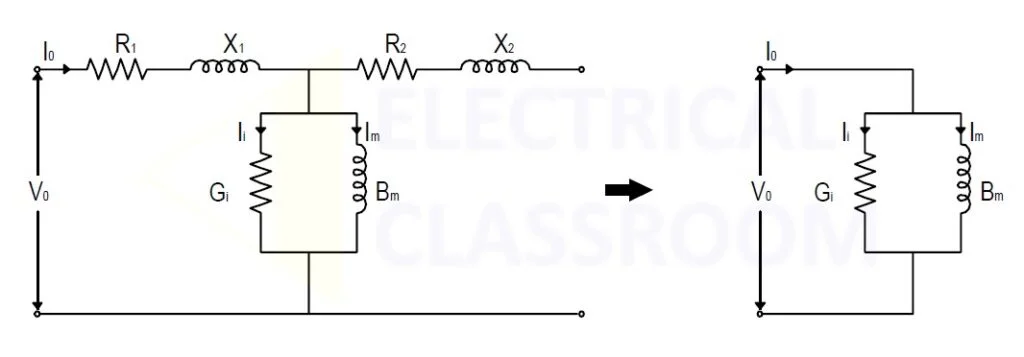# What is magnetization current in a transformer?

A certain amount of current is drawn by a transformer from the source connected to it primary even when no load is connected to its secondary. A part of this current is used to create flux in the transformer core. This current is known as magnetization current.

## Definition of magnetization current

Magnetization current can be defined as “the portion of the no-load current that is used to establish flux in the core of a transformer“. It is generally denoted by the letter Im.

Generally, when a transformer is energized under no-load conditions, it draws a small amount of current. This current is known as excitation current (I0). It is a combination of core loss current (Ii) and magnetization current (Im).

## Properties

• Due to hysteresis, the magnetization current is not sinusoidal.
• Im lags applied voltage by 900.
• Im in a transformer is always constant, irrespective of load.

The no-load test is performed to determine the no-load losses or core losses as well as the turns ratio, no-load currents, magnetization components and core loss components of the transformer.

For convenience, the supply is connected to the LV side of the transformer and the HV side of the transformer is left open. Voltmeters, ammeters and wattmeter are connected as shown in the figure below.

### Procedure

• Apply rated voltage to the LV side of the transformer.
• Measure the no-load current Io, power Po, and input voltage Vo.
• Measure the open-circuited HV side voltage if the transformer ratio needs to be calculated.

### Calculation of core losses and magnetizing componentsAs no load is connected to the secondary, the current flow and the losses due to winding resistance and reactance are very less and can be neglected and the circuit is simplified.

Neglecting the copper loss, we calculate the core losses and the core loss components.Where, Im is the magnetization current and Ii is the core loss component.